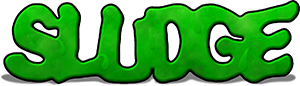## Built-in Constants

If you know anything at all about the inner workings of a computer, you probably know this: numbers are everything. Or more to the point, everything is a number...

In SLUDGE, certain numbers have special meanings. However, the SLUDGE compiler - in a mad effort to be nice to you - lets you type in names instead of these special numbers so that your code makes more sense when you read it. Here, for your viewing pleasure, is a list of (hopefully) all the built-in constants you can use in you SLUDGE code together with what each one actually means.

Remember, you can define your own constants too...

### General constants:

These guys can be found all over the place.

NULL = 0

FALSE = 0

TRUE = 1

NORMAL = 0

### Positioning constants:

Used in a few places... for example addOverlay.

CENTER = 65535

CENTRE = 65535

The following values should only be used by alignStatus.

LEFT = 999

RIGHT = 1001

The following constant has a special meaning if used as a parameter for parallaxAdd.

AUTOFIT = 65535

### Directions:

Directions in SLUDGE are measured in degrees. The following values therefore make for more readable code, for example when you're calling addCharacter or turnCharacter.

NORTH = 0

NORTHEAST = 45

EAST = 90

SOUTHEAST = 135

SOUTH = 180

SOUTHWEST = 225

WEST = 270

NORTHWEST = 315

### Character draw modes:

Used by setCharacterDrawMode, curiously.

TRANSPARENT3 = 1

TRANSPARENT2 = 2

TRANSPARENT = 2

TRANSPARENT1 = 3

DARK1 = 4

DARK2 = 5

DARK = 5

DARK3 = 6

DARK4 = 7

BLACK = 7

FOGGY1 = 11

FOGGY2 = 12

FOGGY = 12

FOGGY3 = 13

FOGGY4 = 14

GREY = 14

GRAY = 14

GLOW1 = 15

GLOW2 = 16

GLOW = 16

GLOW3 = 17

GLOW4 = 18

WHITE = 18

INVISIBLE = 19

### Extra character settings:

Each character can have any combination of these settings... just add them together and throw them through the setCharacterExtra function.

FRONT = 1

FIXEDSIZE = 2

NOZBUFFER = 4

FIXTOSCREEN = 8

NOLIGHTMAP = 16

NOREMOVE = 32

RECTANGULAR = 64

The following constant can be used to turn on the first 5 of the above settings in one fell swoop...

ICON = 31 (i.e. FRONT + FIXEDSIZE + NOZBUFFER + FIXTOSCREEN + NOLIGHTMAP)

### Transition modes:

You'd never guess you used these in transitionMode, would you?

DISOLVE = 1

DISOLVE1 = 1

DISOLVE2 = 2

TVSTATIC = 3

BLINDS = 4

SNAPSHOTBOX = 6

### Speech modes:

Use these and setSpeechMode to turn your game into a full talkie version...

TEXTONLY = 0

SOUNDANDTEXT = 1

SOUNDONLY = 2

### Light map modes:

When you use setLightMap you can also change how lightmaps are to be applied. Use the following values.

HOTSPOT = 0

PERPIXEL = 1